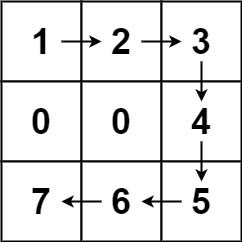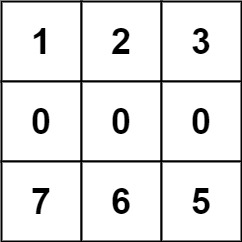# 675.cut-off-trees-for-golf-event

## Statement

• Difficulty: Hard
• Tag: `广度优先搜索` `数组` `矩阵` `堆（优先队列）`

• `0` 表示障碍，无法触碰
• `1` 表示地面，可以行走
• `比 1 大的数` 表示有树的单元格，可以行走，数值表示树的高度``````输入：forest = [[1,2,3],[0,0,4],[7,6,5]]``````输入：forest = [[1,2,3],[0,0,0],[7,6,5]]

``````

``````输入：forest = [[2,3,4],[0,0,5],[8,7,6]]

(0,0) 位置的树，可以直接砍去，不用算步数。
``````

• `m == forest.length`
• `n == forest[i].length`
• `1 <= m, n <= 50`
• `0 <= forest[i][j] <= 109`

• Link: Cut Off Trees for Golf Event
• Difficulty: Hard
• Tag: `Breadth-First Search` `Array` `Matrix` `Heap (Priority Queue)`

You are asked to cut off all the trees in a forest for a golf event. The forest is represented as an `m x n` matrix. In this matrix:

• `0` means the cell cannot be walked through.
• `1` represents an empty cell that can be walked through.
• A number greater than `1` represents a tree in a cell that can be walked through, and this number is the tree's height.

In one step, you can walk in any of the four directions: north, east, south, and west. If you are standing in a cell with a tree, you can choose whether to cut it off.

You must cut off the trees in order from shortest to tallest. When you cut off a tree, the value at its cell becomes `1` (an empty cell).

Starting from the point `(0, 0)`, return the minimum steps you need to walk to cut off all the trees. If you cannot cut off all the trees, return `-1`.

You are guaranteed that no two trees have the same height, and there is at least one tree needs to be cut off.

Example 1:``````Input: forest = [[1,2,3],[0,0,4],[7,6,5]]
Output: 6
Explanation: Following the path above allows you to cut off the trees from shortest to tallest in 6 steps.
``````

Example 2:``````Input: forest = [[1,2,3],[0,0,0],[7,6,5]]
Output: -1
Explanation: The trees in the bottom row cannot be accessed as the middle row is blocked.
``````

Example 3:

``````Input: forest = [[2,3,4],[0,0,5],[8,7,6]]
Output: 6
Explanation: You can follow the same path as Example 1 to cut off all the trees.
Note that you can cut off the first tree at (0, 0) before making any steps.
``````

Constraints:

• `m == forest.length`
• `n == forest[i].length`
• `1 <= m, n <= 50`
• `0 <= forest[i][j] <= 109`

## Solution

``````#include <bits/stdc++.h>
#include <ext/pb_ds/assoc_container.hpp>
#include <ext/pb_ds/tree_policy.hpp>

#define endl "\n"
#define fi first
#define se second
#define all(x) begin(x), end(x)
#define rall rbegin(a), rend(a)
#define bitcnt(x) (__builtin_popcountll(x))
#define complete_unique(a) a.erase(unique(begin(a), end(a)), end(a))
#define mst(x, a) memset(x, a, sizeof(x))
#define MP make_pair

using ll = long long;
using ull = unsigned long long;
using db = double;
using ld = long double;
using VLL = std::vector<ll>;
using VI = std::vector<int>;
using PII = std::pair<int, int>;
using PLL = std::pair<ll, ll>;

using namespace __gnu_pbds;
using namespace std;
template <typename T>
using ordered_set = tree<T, null_type, less<T>, rb_tree_tag, tree_order_statistics_node_update>;
const ll mod = 1e9 + 7;

template <typename T, typename S>
inline bool chmax(T& a, const S& b) {
return a < b ? a = b, 1 : 0;
}

template <typename T, typename S>
inline bool chmin(T& a, const S& b) {
return a > b ? a = b, 1 : 0;
}

#ifdef LOCAL
#include <debug.hpp>
#else
#define dbg(...)
#endif

class Solution {
public:
int n, m;
struct node {
int x, y, v;
bool operator<(const node& other) const {
return v < other.v;
}
};

const inline static int dir[] = {{0, 1}, {0, -1}, {1, 0}, {-1, 0}};

bool ok(int x, int y) {
if (x < 0 || x >= n || y < 0 || y >= m) {
return false;
}

return true;
}

int bfs(int sx, int sy, int ex, int ey, vector<vector<int>>& f) {
auto vis = vector<vector<int>>(n + 1, vector<int>(m + 1, 0));
queue<node> q;
q.push({.x = sx, .y = sy, .v = 0});
vis[sx][sy] = 1;

while (!q.empty()) {
auto front = q.front();
q.pop();

if (front.x == ex && front.y == ey) {
f[ex][ey] = 1;
return front.v;
}

for (int i = 0; i < 4; i++) {
int nx = front.x + dir[i];
int ny = front.y + dir[i];
if (ok(nx, ny) && !vis[nx][ny] && f[nx][ny] >= 1) {
vis[nx][ny] = 1;
q.push({.x = nx, .y = ny, .v = front.v + 1});
}
}
}

return -1;
}

int cutOffTree(vector<vector<int>>& f) {
n = f.size();
m = f.size();

auto tree = vector<node>();
for (int i = 0; i < n; i++) {
for (int j = 0; j < m; j++) {
if (f[i][j] > 1) {
tree.push_back({.x = i, .y = j, .v = f[i][j]});
}
}
}

sort(tree.begin(), tree.end());

int sx = 0, sy = 0;
int res = 0;
for (const auto& o : tree) {
int cur = bfs(sx, sy, o.x, o.y, f);
if (cur == -1) {
return cur;
}

res += cur;
sx = o.x;
sy = o.y;
}

return res;
}
};

#ifdef LOCAL

int main() {
return 0;
}

#endif
``````